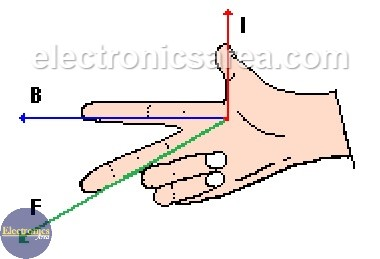# First Right Hand Rule

## First Right Hand Rule – Magnetic Force induced on a Current-Carrying Wire.

John Ambrose Fleming, a British Physicist and electronic engineer invented the First Right Hand Rule to find the direction of the magnetic field produced by an electric current.

The First Right Hand Rule or (Magnetic Force induced on a Current-Carrying Wire) says that if a current-carrying wire is in the presence of a magnetic field, a force is exerted on the wire. This force has the formula: F = iBLsenα.

Where:

i = current flowing through the wire
B = magnetic field
L = wire length
α = angle between the current direction flow and the the magnetic field direction.

## First Right Hand Rule ExplanationFirst Right Hand Rule – Magnetic Force induced on a Current-Carrying Wire.

See the previous image.

“If a current (i) flows in the direction shown by the thumb and the magnetic field (B) has the direction shown by the index finger, it is exerted on the wire that carries the current (i), a force (F) that has the direction shown by the middle finger. ”

The directions of the current (i), the magnetic field (B) and the force (F) are perpendicular.

The above case is explained for a single conductor wire. In the case of N conducting wires in the presence of a magnetic field, the total magnetic force induced is the force exerted on a wire multiplied by N.

Then the new formula is: F = NiBLsenα.

• 5
•
•
•
•
•
•
•
•
5
Shares
•
5
Shares
• 5
•
•
•
•
•
•
•